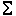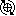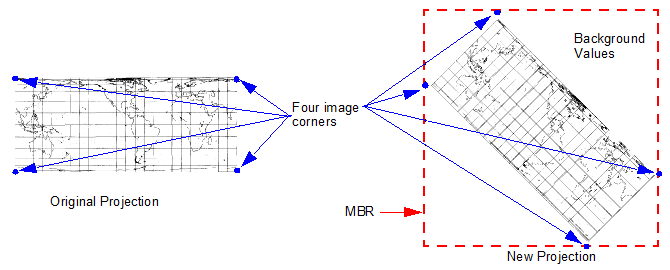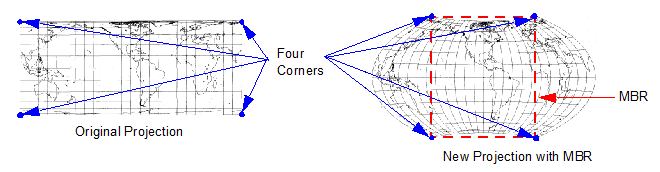# Transformation Matrix

## ERDAS IMAGINE Help

HGD_Variant
16.5.1
HGD_Product
ERDAS IMAGINE
HGD_Portfolio_Suite
Producer

A transformation matrix is a set of numbers computed from the GCPs that can be plugged into polynomial equations. These numbers are called the transformation coefficients. The polynomial equations are used to transform the coordinates from one system to another.

The transformation coefficients are reported in a CellArray in the Transformation tab in the Polynomial Model Properties dialog. The following are discussed in this topic:

• Manually Calculate the Transformation Matrix
• Automatically Calculate the Transformation Matrix
• Minimum Number of GCPs
• Residuals and RMS Error Units
• Direction of Transformation
• Reprojection Grid and Minimum Bounding Rectangle

Manually Calculate the Transformation Matrix

To calculate a transformation matrix on a GCP by GCP basis, click the Solve Geometric Model with Control Points buttonin the Multipoint Geometric Correction workspace after collecting a GCP.

Base Calculations on All or Specific GCPs

You can base your calculations on all the GCPs in the CellArray, or on specific selections in the CellArray. Selected GCPs are calculated only on the control points.

Your selection may change as you view the RMS error and contribution of each point, as shown in the right side of the GCP CellArray.

Automatically Calculate the Transformation Matrix

To compute the transformation in real time as you edit the GCPs or change the selection in the CellArray, click the Set Automatic Transformation Calculation button.

When the Set Automatic Transformation Calculation button is active, you can move a GCP in the Multipoint Geometric Correction workspace while watching the transformation coefficients change on the Transformation tab in the Polynomial Model Properties dialog.

Minimum Number of GCPs

Higher orders of polynomial transformation can be used to correct more complicated types of distortion. However, to use a higher order of transformation, more GCPs are needed. For the best rectification results, you should always collect more than the minimum number of GCPs, and the GCPs should be well-distributed and as precise as possible.

If the minimum number of points is not satisfied, then an error message dialog opens. The coefficients in the Transformation tab, RMS errors, and residuals are blank. At this point, you will not be allowed to save the transformation or resample the data.

To change the order of the transformation, click the Parameters tab in the Polynomial Model Properties dialog.

Residuals and RMS Error Units

The transformation matrix contains the coefficients for transforming the reference coordinate system to the input coordinate system. Therefore, the units of the residuals and RMS errors are the units of the input coordinate system.

Direction of Transformation

By default, the transformation matrix in the reference-to-input transformation converts reference coordinates to the input coordinate system. Although this may sound backwards, this is the transformation that is actually used in the resampling process.

Reprojection Grid and Minimum Bounding Rectangle

The Reprojection Model Properties dialog provides a shortcut for reprojecting data from one map projection system to another.

By this method, the four corners of the image are taken and converted into the new projection system. Then the Minimum Bounding Rectangle (MBR) containing those corners is calculated.

Generating the MBRt

Input GCPs are generated automatically from a grid on the source data that you specify, and the corresponding reference GCPs are computed from map projection data that you provide. These GCPs are derived and used transparently. They do not appear in the Multipoint Geometric Correction workspace, nor are they stored to a file.

In a very small number of cases, the MBR may actually cut off part of the image.

Generating the MBR omits part of the imageThere are two ways to solve this.

• Use the rigorous Reproject Images dialog to reproject the image.
• In the Resample dialog, you can click the Recalculate Output Defaults button and significantly decrease the Skip Factor X and Skip Factor Y values. This will ensure that the entire image is used to calculate the image extent.

In Reproject Model Properties dialog, you enter Grid Sampling X and Grid Sampling Y, which is the number of GCPs in the X and Y dimensions of a grid. For example, if you specify a grid sampling density of 8 in the X direction and 8 in the Y direction, there will be 64 GCPs (8 x 8 = 64) sampled in a grid pattern across the source data. (You do not see this grid in the View.)

From those GCPs, a transformation matrix is calculated. RMS error information displays in the Reprojection Model Properties dialog. You can use the transformation coefficients to create an output image using the Resample dialog.